## Determining the internal resistance of a given primary cell using cell using potentiometer

Aim : To determine the internal resistance of a given primary cell using cell using potentiometer .

Apparatus : a potentiometer , a battery , (or eliminator ) , two one way key , a rheostat of low resistance , a galvanometer , a high resistance box , a fractional resistance box , an ammeter , a voltmeter , a cell , a jockey , a set square , connecting wires , a piece of sand paper .

Theory

When Key K2 is open and K1 is closed ,

Let null point be obtained at a distance l1 from A

: E =Kl1 (1)

When key K2 is closed and K1 is open ,

Let null point be obtained at a distance l2 from A

V=Kl2 (2)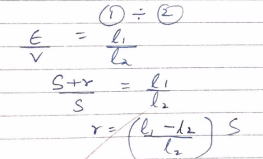Where S is the shunt resistance in parallel with given cell .

l1 and l2 ; balancing length without & with shunt respectively .

r: internal resistance of the cell.

Procedure

Make the connection as shown in diagram .

Clean the ends of the connecting wires with sand paper and make tight connection , tighten the plug of the resistance box .

Check the emf of the battery and cell and see the emf of the battery is more than that of the given cell other wise null or balance point wont be obtained (E` >E).

Take maximum current from battery , making rheostat resistance small.

Insert the plug key k , and adjust the rheostat so that a null point is obtained on the fourth wire of the potentiometer .

Insert the 2000 ohm plug in its position in resistance box and obtain a null point by slightly adjusting the jockey .

Measure the balancing length l1.

Take out the 2000 ohm plug from the resistance box . introduce the plug in the key k1 as well in key k2 .Take out a small resistance from the resistance box R connected in parallel with cell.

Slide the jockey along the potentiometer wire and obtain a null point

Insert the 200 ohm plug back in its position in RB and make further adjustment for sharp null point .

Measure the balancing length l2 from end P.

Remove the plugs key k1 and K2 . wait for some time and repeat the activity for the same current .

Circuit Diagram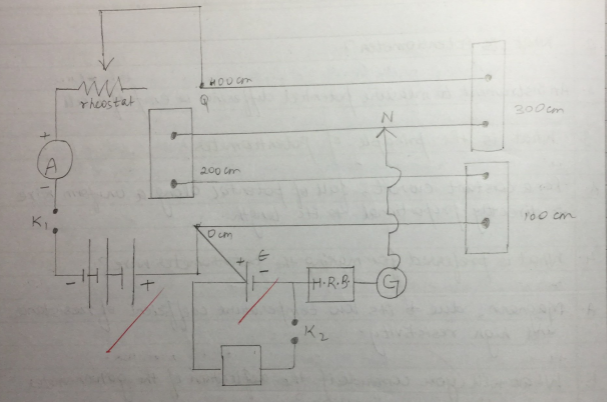Observation

 Value of Shunt resistance (S in ohm) Balance Length l1 (K2 is open) without Shunt (cm) Balance length l2 with Stunt (K2 is closed) (cm) r = [(l1-l2)S]/l2 (in ohm) Mean ‘r’ 1.5 171.4 64 1.67 1.77 ohm 2 171.3 61.5 1.78 2.5 171.1 59.6 1.87

Calculation

Mean ‘r’ = (1.67+1.78+1.87)/3 = 1.77 ohm

Result

The internal resistance (R) of given cell is 1.77 OHM

Precaution

For one set of observation the ammeter reading should be constant .

Current should be passed for short time .

Jockey should be rubbed against potentiometer wire .

Sources of error

The emf of the battery is less then the cell (E`<E)

Cell is disturbed during the experiment .

Viva Questions

What do you mean by the internal resistance of cell ?

It is the resistance offered by electrodes

What are the factors on which internal resistance of a cell depends ?

1 Nature of electrodes

2 Nature of electrolyte

3 concentration of electrolyte

4 Temperature of electrolyte

5 Distance between electrodes

6 Area immersed

Does the internal resistance depends on current drawn from the cell ?

Yes the internal resistance usually increases as more current is drawn fom the cell

Can we find the internal resistance of a secondary cell ?

No the internal resistance of a secondary cell is so small (0.01 ohm ) that this method can’t be used .

What should a cell not be disturbed during the experiment ?

Disturbing the cell may change the factors on which internal resistance depends

You can also get Class XII Practicals on BiologyPhysics, and Physical Education.

## Determining resistance of a galvanometer by half deflection method and to find its figure of merit .

Aim : To determine resistance of a galvanometer by half deflection method and to find its figure of merit .

Apparatus : A Weston type of galvanometer , a voltmeter , a battery /battery eliminator , two 10,000 ohm and 200 ohm ) resistance boxes , two one way key , a rheostat , a screw gauge , a meter scale , an ammeter of given range , connecting wires and a piece of sand paper .

Theory

The resistance of the given galvanometer as found by half deflection method .

G= (RxS)/(R-S) (1)

Where R is the resistance connected in series with the galvanometer and S is the shunt resistance .

The figure of merit , K =E /[(R+G)Q] (2)

Where E is the end of the cell and Q is the deflection produced with resistance R.

The maximum current that can pass through the galvanometer , Ig=nk

Where n is the total number of dimension on the galvanometer scale on either side of zero .

Procedure

1. Resistance of galvanometer by half deflection method

• Make the connections accordingly as shown in circuit diagram .
• See that all plugs of the resistance boxes are tight
• Take out the high resistance (200 Ohm ) from the resistance box R and insert key K1 only .
• Adjust the value of R1 so that deflection is maximum , even in number and within the scale .
• Note the deflection . Let it be Q.
• Insert the key k2 also and without changing the value of R2 ,adjust the value of S , such that deflection in the galvanometer reduces to exactly half the value of resistance S.
• Note the value of resistance S .
• Repeat step 4 & 7 three times taking different R and adjusting .

2. Figure of merit

• Take one cell of the battery and find its emf by a voltmeter by connecting +ve of the voltmeter with +ve of cell and – ve of the voltmeter with -ve of cell . Let it be E.
• Take connection as in circuit diagram .
• Adjust the value of R to obtain a certain deflection Q when circuit is closed .
• Note the value of resistance R and deflection Q.
• Now change the value of R and note the galvanometer deflection again .
• Repeat the step with both cells of the battery with different voltages like 2,4,6 ,8 volts from battery eliminator.
• Find the figure of merit K using the formula

Observation table

 Resistance (R) Deflection (Q) Shunt (S) G =(RxS)/(R-S) 5000 10 5 5.005 10000 6 3 3 2000 20 10 10.05 1500 26 3 13.11 Average G = 7.79 ohms
 Resistance (R) Deflection (Q) K 5000 9 0.556 10000 5 0.60 2000 20 0.502 1500 25 0.524 Average K = 0.5455 A/dn

Calculations

1. G = (5.005+3+10.05+13.11)/4 [E = 1.45V]
2. Figure of Merit : Current in the galvanometer per unit time
3. K = IQ = [(E)/(R+G)]x[1/Q] – SI unit = AD division = 10-5 Al div

Result

Resistance of given galvanometer is 7.79 ohm .

Figure of merit of given galvanometer is 0.545 A/dn .

Precautions

All connections should be neat and tight .

The emf of cell / battery should be constant .

Source of error

The scene of the instrument may be loose .

The galvanometer division may not be of equal size.

Diagram

Resistance of Galvanometer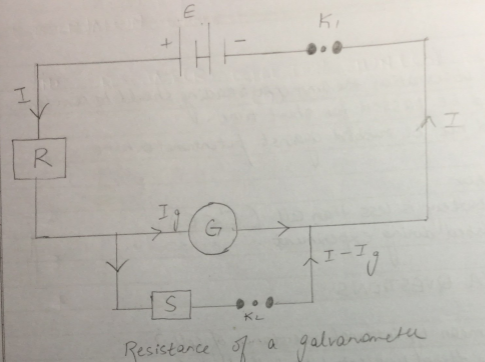Figure of Merit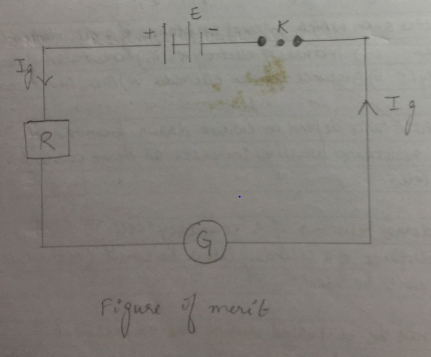Viva question

What is the galvanometer ?

It is a device used for detecting feeble electric currents in circuit .

Define the figure of merit of a galvanometer ?

The quantity of current required to produce a deflection of one division in the galvanometer is called figure of merit of a galvanometer . It is represented by K . Its unit is ampere per division .

Why is this method called half deflection method ?

It is so because the deflection is made half by using a shunt resistance S.

Under what condition id the resistance of a galvanometer (E1) equal to shunt resistance (S)?

E1=S , only when series resistance R is very high .

You can also get Class XII Practicals on BiologyPhysics, and Physical Education.

## To compare EMF of two given primary cells using potentiometer

Aim : To compare EMF of two given primary cells using potentiometer

Apparatus : potentiometer , a leclanche cell , a daniel cell , an ammeter , a voltmeter , a galvanometer , a battery , (battery eleminator), a rheostat , of low resistance , a resistance box , a one way key , a two way key , a jockey , a set square , connecting wire , a piece of sand paper .

Theory

When we keep key (K1) closed and (K2) open , let the null point found be lAJ1

E1=KlAj1 (1)

When we keep K1 open and K2 closed , let null point obtained by lAJ2 .

E2=KlAj2 (2)

(1)/ (2)

E1 / E2 = KlAJ1 / KlAj2

E1/E2 =l1/l2

Where E1 and E2 are the emf of two given cells .

Procedure

1. Arrange the apparatus as shown in the circuit diagram .
2. Connect the positive poles of the cells to the terminal and the negative poles to the terminal a and b of the two way key .
3. Insert the plug in the key K and also in between the terminals a and c of the two way key .
4. Slide the jockey gently over the potentiometer wires until you obtain a point of no deflection .
5. Note the length l1 at the point.
6. Repeat this with E2 by disconnecting E1 and inserting plug into gap a and c of two way key .
7. Record l2 at null point .
8. Repeat this different resistance .

Observation table

 Balancing Lengths E1 / E2 = lAJ1 / lAj2 L1 for cell E1 (cm) L2 for cell E2 (cm) 327 376 0.86 323.5 371 0.87 321.5 369 0.87 312.5 352.5 0.88

Result

The ratio of emf E1/E2 ~0.87

Precaution

1. The connections should be neat, clean and tight.
2. The plugs should be introduced in the keys only when the observations taken.
3. The positive poles of the battery E and cells Ex and E2 should, all be connected to terminal at the zero of the wires.
4. The jockey key should not be rubbed along the wire. It should touch the
5. The e.m.f. of the battery should be greater than the e.m.f.’s of the either cells.
6. Some high resistance plug should always be taken out from resistant the jockey is moved along the wire.

Sources of error

1. The auxiliary battery may not be fully charged.
2. The potentiometer wire may not be of uniform cross-section and material. throughout its length.
3. End resistances may not be zero.

Diagram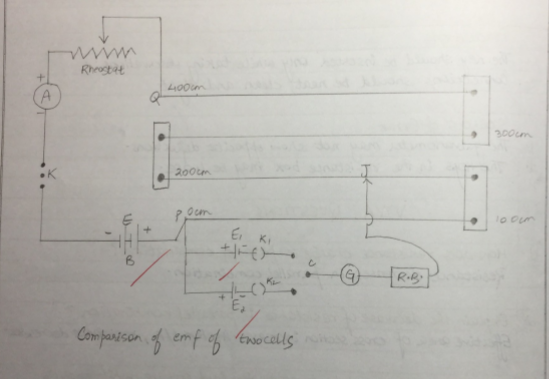VIVA Question

What is emf of a cell?

Electromotive force of a cell is the potential difference across the terminals of the cells when it is in open circuit i.e. no current is drawn from the circuit .

What is potentiometer ?

An instrument to measure potentiometer difference or emf of a cell .

What is the principle of potentiometer ?

For a constant current , fall of potentiometer along a uniform wire is directly proportional to its length .

What is preferred for making potentiometer wire ?

Magnum , due to its low temperature coefficient of resistance and high resistivity .

What will you conclude if the deflection of the galvanometer is in the same direction ?

The positive terminals aren’t connected at one point

Potential difference between wires ends is less than emf of cells to be measured .

Emf of driving cells is less than that of cells to be measured .

You can also get Class XII Practicals on BiologyPhysics, and Physical Education.

## To verify the laws of combination (series) of resistance using a meter bridge (R=R1 + R2 )

Aim : To verify the laws of combination (series) of resistance using a meter bridge (R=R1 + R2 )

Apparatus : a meter bridge , a leclanche cell (battery eliminator ) , a galvanometer , a resistance box , a jockey , two resistance wire or two resistance coils , set square , connecting wires .

Theory

1. The resistance (r) of a wire or coil is given by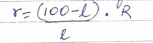Where R is the resistance from resistance box in the left gap and l is the length of the meter bridge wire from zero end upto balance point .

2. When two resistance r1 & r2 are connected in series , then their combined resistance

R3=R1+R2

Procedure

1. Mark the two resistance coil as r1 and r2 .
2. To find the r1 and r2 proceed same way as in experiment 1.

(if r1 and r2 are not known )

1. Connect the two coils r1 and r2 in series as shown circuit diagram in the right gap of meter bridge and find the resistance of this combination. Take at least three sets of observation.

Result

Within limits of experimental error , experimental and theoretical values of R  are same . Hence law of resistance in series is verified .

Precaution

1. The key should be inserted only while taking observation .
2. Connections should be neat and tight .

Sources of error

1. The key in resistance box may be loose .
2. The galvanometer may not show opposite deflection .

Circuit diagram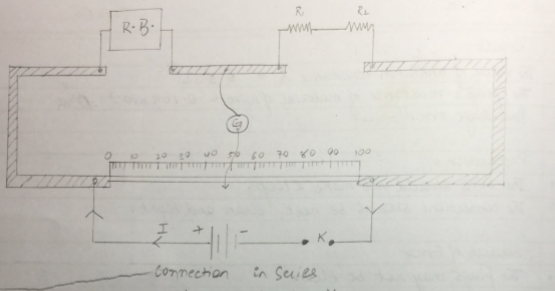Observation table

 Resistance in R.B. (ohm) Balance l (cm) (100-l) (cm) [(100-l)*R]/l Mean r1 (cm) 0.5 24 76 1.583 1.616 1 38 62 1.631 2 55 45 1.636
 Resistance in R.B. (ohm) Balance l (cm) (100-l) (cm) [(100-l)*R]/l Mean r2 (cm) 0.5 33 67 1.015 1.015 1 50 50 1 2 66 34 1.030
 Resistance in R.B. (ohm) Balance l (cm) (100-l) (cm) [(100-l)*R]/l Mean rs (cm) 0.5 16 84 2.625 2.615 1 28 72 2.571 2 43 57 2.651

Calculation

R1 + R2 = 1.616+1.015 = 2.631 ohm
Rs = 2.615 ohm

Therefore, experimental error = [(2.615-2.631)/2.615]*100 = 0.6%

Viva question

How does resistance change in series combination ?

Ans. Resistance increase in series combination

Why does resistance increase in series combination ?

Ans. Effective length of resistor increases . As R*l , resistance increases .

What is resistance ?

Ans. it is the opposition offered by the material of two wire to flow of electric current .

What is the cause of resistance ?

Ans. it is the opposition offered by the material of wire to flow of electric current .

What is the effect of temperature In the resistance of conductor ?

Resistance of all conductor increases with increase in temperature .

You can also get Class XII Practicals on BiologyPhysics, and Physical Education.

## Verifying laws of combination (parallel) of resistance using meter bridge

Aim: To verify laws of combination (parallel) of resistance using meter bridge .

Apparatus: a meter bridge , a leclanche cell , a galvanometer , a jockey , a jockey , a resistance box , two resistance wire , connecting wires , set square .

Theory

The resistance (r) of a resistance wire or coil is given by r=(100-l)/L *R where R is the resistance from resistance box in the left gap and L is the length of meter bridge wire from O end upto balance point.

When r1 and r2 are connected in parallel , then their combined resistance

Rp = r1r2 /r1+r2

Procedure

1. Mark the two resistance wires as r1 and r2
2. Connect the two coils r1 and r2 in parallel as shown in fig. in the right gap of meter bridge and find the resistance of this combination . Take at least three set of observation

Result

Within limit of experimental error , experimental and theoretical values of Rp are same .

Hence law of resistance in parallel are verified .

Precaution

The key should be inserted only while taking observation .

Connection should be neat and tight .

Source of error

The galvanometer may not show opposite deflection

The key in the resistance box may be close .

OBSERVATION TABLE

Value of r1

 Resistance of R.B. (ohm) Balance L (cm) (100-l) (cm) [(100-l)*R]/L Mean r1 0.5 59 41 0.347 0.6393 1 37 63 0.587 2 33 67 0.985

Value of r2

 Resistance of R.B. (ohm) Balance L (cm) (100-l) (cm) [(100-l)*R]/L Mean r2 0.5 83.2 16.8 0.1009 1.729 1 80.8 19.2 4.208 2 69.4 30.6 0.881

Value of rp

 Resistance of R.B. (ohm) Balance L (cm) (100-l) (cm) [(100-l)*R]/L Mean rp 0.1 27 73 0.27 0.36 0.2 32 68 0.42 0.5 56 44 0.39

Calculations

Rp = (R1R2)/R1+R2 = (0.693×1.729)/(0.693+1.729) = 0.4667 ohm

Experimental Error = [(0.36-0.4667)/(0.36)]*100 = 29.6%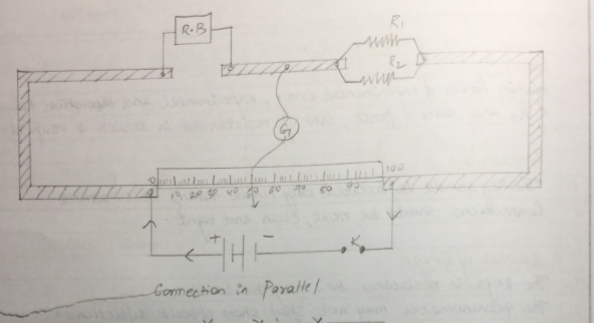Viva question

1. How does resistance change in parallel combination ?

Resistance decrease in parallel combination

2. explain the decrease of resistance in parallel combination ?

Effective area of cross section increases as r x 1/A , resistance decreases .

3. Why should jockey not be pressed too hard on wire while sliding ?

Sliding the jockey with a hard press will scratch the wire and make its thickness non-uniform .Resistance per unit length of wire will be inconsistent as resistance depends on area of cross section .

4. What is the resistance of an open key ? Explain.

Open key has infinite resistance as it makes current zero (R=V/I)

5. Define unit of resistance .

SI unit of resistance is OHM or volt per ampere . 1 Ohm is the resistance of a conduction carrying 1 ampere current when potential difference maintained across its end is 1 volt .

You can also get Class XII Practicals on BiologyPhysics, and Physical Education.

## Finding the resistance of a given wire using meter bridge and hence determine resistivity of its material

Aim – To find the resistance of a given wire using meter bridge and hence determine resistivity of its material .

Apparatus

A meter bridge (slide wire bridge ) , a lecranche cell, a galvanometer , a resistor , a jockey , a one way key , a resistance wire , a screw gauge , a meter scale , a set of square , connecting wire , a piece of sand paper .

Theory :

The unknown resistance ‘X” is given by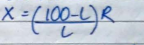1. Where ‘R” is the known resistance placed in the left gap & unknown resistance ‘X” is the right gap of meter bridge .’L’ is length of meter bridge wire from zero end upto balance .
2. Resistivity of the material of the given wire is given by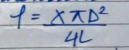Where ‘L’ is the length & D is the diameter of the given wire .

Procedure

1. Arrange the apparatus as shown in the arrangement diagram .
2. Connect the resistance wire whose resistance is to be determine in the right gap b/w C& B .Take care that no point /part of the wire forms a loop.
3. Connect resistance box of low range in the left gap b/w A & B .
4. Make all other connection as shown in the circuit diagram .
5. Take out some resistance from the resistance box , ping the key ‘K’
6. Touch the jockey gently first at length end & then right end of the bridge wire .
7. Note the deflection in the galvanometer. If the galvanometer shows deflection in the galvanometer reading in opposite direction the correction are correct. If the deflection is on one side only then there is fault in the circuit . Check & rectify the fault .
8. Move the jockey gently along the wire from left to right till gives zero deflection . The point where the jockey is touching the wire is null point ‘D’.
9. Choose an appropriate value of ‘R’ from the box such that there id no defection in the galvanometer when the jockey is nearly in the middle of the wire .
10. Note position of point ‘D’ to known the length AD=l
11. Take atleast 4 sets of observation in the same way by changing the value of R in the steps .
13. For specific resistance
1. Cut the resistance wire at the point where it leaves the terminal , started it & find its length by using a meter scale .
2. Measure the diameter of the wire at least at 4 places in two mutually perpendicular direction at each place with the of screw gauge .
3. Record your observation as given in the table .

Observation

1. Length of a given wire L = 66 cm =0.66 m
2. Table for unknown resistance (X)
 Resistance from box, R (Ohm) Length AB =l (cm) Length BC = (100-l) (cm) Unkown Resistance X = [R(100-l)]/L (ohm) 0.5 58.3 41.7 0.35 0.7 60.7 39.3 0.45 1 61.9 38.1 0.61 1.5 61.1 38.9 0.95 Mean = 0.59

3. Least count of screw gauge

Pitch of screw gauge =0.01

Total no of division on the circular scale =

LC of screw gauge = Pitch /No of the circular scale

Zero error (e) =(0)

Zero connection =(e)=0

 Main Scale Reading (mm) Circular Scale Reading Total Reading (diameter) (mm) Mean D (mm) Mean radius (D/2) (mm) 0 43 0.43 0.42 0.21 0 41 0.41

Result

1. The value of the unknown resistance X =0.5 ohm
2. The specific resistance of material of wire = 0.104 x10-3 ohm m
3. Percentage error

Precaution

The connection should be neat, clean & tight.

Source of error

Plug may not be clean

The wire may not be of uniform thickness.

Viva questions

1. Why is the meter bridge so called?

Since the bridge uses one meter long wire, it is called a meter bridge .

1. What is a null point ?

It is a point on the wire , keeping jockey at which galvanometer gives 0 deflection .

1. Why is the bridge method better that ohms law for measurement ?

It is so because the bridge method is a null method (at null point , no current is flowing in galvanometer ) and more sensitive .

1. Why copper strips used to press ends of wire are thick ?

Thick Cu strips have negligible resistance over the resistance of alloy meter bridge wire and minimize affect of end resistance .

Circuit diagram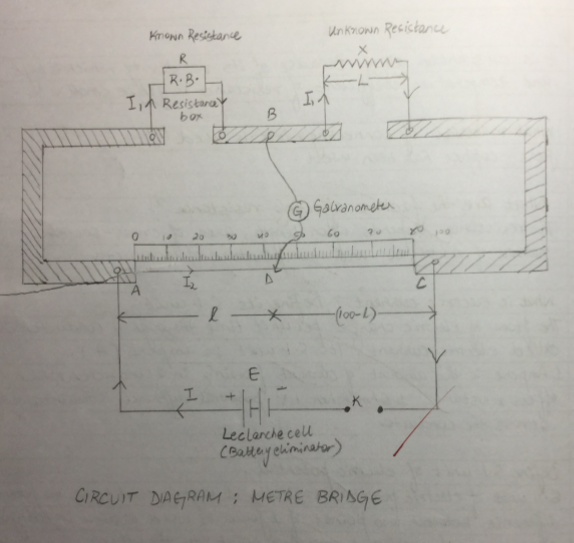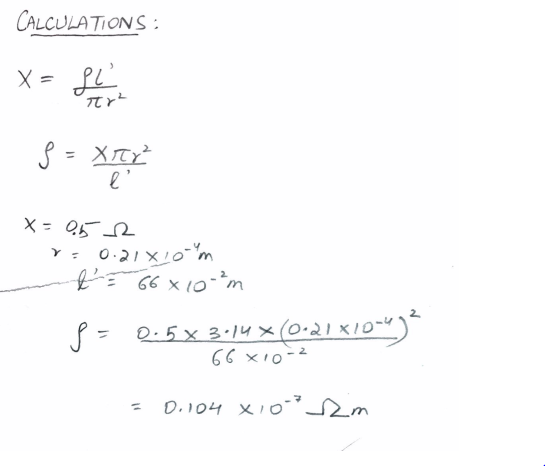You can also get Class XII Practicals on BiologyPhysics, and Physical Education.

## Internal Resistance Of a Given Primary Cell Using a Potentiometer

OBJECTIVE

To determine the internal resistance of a given primary cell using a potentiometer.

APPARATUS

A potentiometer, a battery (or battery eliminator), two one-way keys, an id resistance, a galvanometer, a high resistance box, a fractional resistance box, an ammeter,  voltmeter, a cell (say Leclanche cell), a jockey, a set square, connecting wires and sandpaper.

CIRCUIT DIAGRAM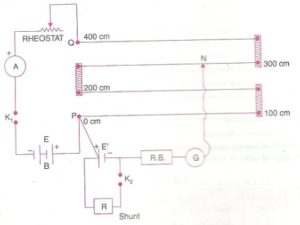THEORY

When Key K2 is open and K1 is closed,
Let null point be obtained at a distance l1 from A
Therefore E = Kl1 –> (1)
When Key K2 is closed and K1 is open,
Let null point be obtained at a distance l2 from A
Therefore V = Kl2 –> (2)
(1) divided by (2)
E/V = l1/l2
(S+r)/S  = l1/l2
r = S*(l1-l2)/l2

Where S : the shunt resistance in parallel with given cell.
l1 and l2 : Balancing lengths without and with shunt respectively.
r : internal resistance of the cell.

PROCEDURE

1. Make the connections as shown in circuit diagram.
2. Clean the ends of the connecting wires with sandpaper and make tight connections. Tighten the plugs of the resistance box.
3. Check the E.M.F. of the battery and cell and see that E.M.F. of the battery is more than that of the given cell otherwise null or balance point won’t be obtained. (E’ > E)
4. Take maximum current from the battery, making rheostat resistance small.
5. Insert the plug in Key K1 and adjust the rheostat so that a null point is obtained or the fourth wire of potentiometer.
6. Insert the 2000 Ohm plug in its position in resistance box and obtain a null point by slightly adjusting the jockey.
7. Measure the balancing length L1.
8. Take out the 2000 Ohm plug from the resistance box. Introduce the plugs in Key K1, as well as in Key K2. Take act a small resistance from the resistance box R connected in parallel with the cell. Slide the jockey along the potentiometer wire and obtain a null point. Insert the 2000 Ohm plug back in its position in R.B. and make a further adjustment for a sharp null point.
9. Insert the 2000 Ohm plug back in its position in R.B. and make a further adjustment for a sharp null point.
10. Measure the balancing length l2 from end P.
11. Remove the plugs keys K1 and K2. Wait for some time and respect the activity for the same current.

OBSERVATIONS

• Range of voltmeter
•  Least count of voltmeter
•  E.M.F. of battery (or battery eliminator)
•  E.M.F. of cell

CALCULATIONS

RESULT

The internal resistance of the given cell is…………… ohm.

PRECAUTIONS

1. The e.m.f. of the battery should be greater than that of the cell.
2. For one set of observation, the ammeter reading should remain constant.
3. Current should be passed for a short time only while finding the null point.

You can also get Class XII Practicals on BiologyPhysics, and Physical Education.

## Determining resistance per cm of given wire by plotting a graph of potential difference versus current

AIM To determine the resistance per cm of a given wire by plotting a graph of potential difference versus current.

APPARATUS REQUIRED
Battery, key, rheostat, voltmeter, ammeter, resistance wire (unknown resistance), connecting wires, meter scale, sandpaper.

PRINCIPLE
This Experiment is based on OHM’S LAW
Ohm’s Law states that the electric current passing through a conductor is directly proportional to the potential difference applied across its ends.
Mathematically, V=IR
The resistance R of the wire depends on the material of the wire and its dimensions.

CIRCUIT DIAGRAM

PROCEDURE

1. Draw the circuit diagram as shown in figure above
2. Arrange the apparatus as per the circuit diagram
3. Clean the ends of the connecting wires with sandpaper and make them shiny.
4. Make the connections as per circuit diagram. All connections must be neat and tight. Take care to connect the ammeter and voltmeter with their correct polarity. (+ve to +ve and -ve to -ve)
5. Determine the zero error and least count of the ammeter and voltmeter and record them.
6. Adjust the rheostat to pass a low current.
7. Insert the key K and slide the rheostat contact to see whether the ammeter and voltmeter are showing deflections properly.
8. Adjust the rheostat to get a small deflection in ammeter and voltmeter.
9. Record the readings of the ammeter and voltmeter
11. Plot a graph with V along X axis and I along axis.
12. The graph will be a straight line which verifies Ohm’s law
13. Determine the slope of the V-I graph. The reciprocal of the slope gives the resistance of the wire.

OBSERVATIONS

1. Range
Range of the given Ammeter =  0-500m A
Range of the given voltmeter =  0-5V
2. Least Count
Least Count of the given Ammeter = 10mA
Least Count of the given voltmeter = 0.1V
3. Zero Error
Zero Error of the given Ammeter = 0A
Zero Error of the given voltmeter = 0V
4. Zero Correction
Zero Correction of the given Ammeter = 0A
Zero Correction of the given voltmeter = 0V
5. Observation Table for Ammeter and Voltmeter Readings
 S No Ammeter Observed (A) Ammeter Corrected (A) Voltmeter Observed (V) Voltmeter Corrected (V) Ratio (V/I) = R (ohm) 1 0.3 0.3 0.1 0.1 0.33 2 0.7 0.7 0.2 0.2 0.28 3 1.1 1.1 0.3 0.3 0.27 4 1.5 1.5 0.4 0.4 0.26 5 1.9 1.9 0.5 0.5 0.26

Graph

CALCULATIONS
Mean Value of V/I from observations, R = 0.28Ω
Length of resistance wire = 40.2cm
Value of slope of VI graph =0.27 Ω
Resistance per unit length = 0.675 Ωm-1

RESULT

1. Ohm’s Law is verified as the I vs V graph is a straight line
2. The resistance of the given wire = 0.28Ω
3. The resistance per cm of given wire is 0.675 Ω m-1.

PRECAUTIONS

1. All the electrical connections must be neat and tight.
2. Voltmeter and Ammeter must of proper range
3. The key should be inserted only while taking readings.

VIVA VOCE

• Give the mathematical form of Ohm’s law.
V = RI,  is the mathematical representation of Ohm’s law.
• State Ohm’s Law
Ohm’s law states that the electric current ‘I’ flowing through a conductor is directly proportional to the potential difference V (voltage) across its ends (provided that the physical conditions, temperature, pressure, and dimensions of the conductor remain same).
• What is the material chosen for rheostat wire and why?
It is constantan alloy. It is chosen to make rheostat as its resistivity is very high and temperature coefficient of resistance is quite small.
• What is the material used for making connection wires?
Here, copper has been used.
• What are the factors affecting resistance?
The resistance depends upon length, the area of cross-section, nature of material and temperature of conductors.
• What is electrical current? Define its S.I. unit?
The flow of electric charge per unit time through a conductor is called the electric current. It’s S.I. unit is ampere (A). 1 Ampere is the amount of current flowing in a conductor which offers resistance 1 Ohm when 1 V potential difference is maintained across the conductor.
• Define S.I. Unit of electric potential.
S.I. unit of electric potential is Volt. 1 Volt is said to be the potential difference between two points if 1 joule of work is done in bringing 1 coulomb of charge from one point to the other.
• Why is ammeter connected in series?
To measure the current without any change in magnitude.
• Why is voltmeter connected in parallel?
A voltmeter is connected in parallel so that it can measure the potential drop without any change in its magnitude.

You can also get Class XII Practicals on BiologyPhysics, and Physical Education.